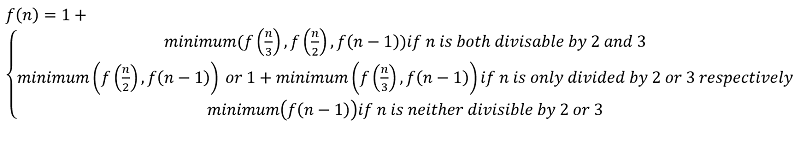Q:

# Minimum steps to minimize n as per given condition

Given a number n, count minimum steps to minimize it to 1 performing the following operations:

1. Operation1: If n is divisible by 2 then we may reduce n to n/2.
2. Operation2: If n is divisible by 3 then you may reduce n to n/3.
3. Operation3: Decrement n by 1.

Input:

Input is a single integer, n.

Output:

Output the minimum number of steps to minimize the number performing only the above operations.

Constraints:

```1 <= N <= 10000
```

Example:

```Test case 1:
Input:
N=10

Output:
Minimum number of steps needed: 3

Test case 2:
Input:
N=6

Output:
Minimum number of steps needed: 2
```

Explanation:

```For the above test case,
N=10

N is not divisible by 3, but by 2
So,
10->5
Now % is again neither divisible by 3 nor 2
So, only option is to decrement

Hence
5->4
4 can be decremented to 2 by dividing by 2
4->2
2->1

So,
The conversion path will be
10->5->4->2->1
Total 4 steps
But is this the optimal one?

The optimal will be
10 converted to 9 by decrementing
10->9
9->3->1

So, total of 3 steps which is the minimum number

The above problem is a classic recursion example.

Like,

1. If n is divided by both 2 and 3
Recur for possibilities (n/3), (n/2), (n-1)
2. If n is divided by only 3 but not by 2 then
Recur for possibilities (n/3), (n-1)
3. If n is divided by only 2 but not by 3 then
Recur for possibilities (n/2), (n-1)
4. If n is divided by only 3 but not by 2 then
Recur for possibilities (n/3), (n-1)
5. If n is not divisible by both 2 and 3
Then only recur for (n-1)

We can write all this with help of recursive function,

```Let,
f(n) = the minimum number of steps to convert n to 1
```If you draw the recursion tree for f(10) you will find many overlapping sub-problems. Hence, we need to store all the already computed sub-problems through memorization.

```Function minimumSteps(n)
if(n==1)
return 0;

// memoization here, no need to compute if already computed
if(dp[n]!=-1)
return dp[n];
// store if not computed
if(n%3==0 && n%2==0)
dp[n]=1+min(minimumSteps(n-1),min(minimumSteps(n/3),minimumSteps(n/2)));
else if(n%3==0)
dp[n]=1+min(minimumSteps(n-1),minimumSteps(n/3));
else if(n%2==0)
dp[n]=1+min(minimumSteps(n-1),minimumSteps(n/2));
else
dp[n]=1+minimumSteps(n-1);
end if
return dp[n]
End Function
```

C++ Implementation:

``````#include <bits/stdc++.h>
using namespace std;

int dp;

int minimumSteps(int n)
{

if (n == 1)
return 0;

if (dp[n] != -1)
return dp[n];

if (n % 3 == 0 && n % 2 == 0) {
dp[n] = 1 + min(minimumSteps(n - 1), min(minimumSteps(n / 3), minimumSteps(n / 2)));
}
else if (n % 3 == 0) {
dp[n] = 1 + min(minimumSteps(n - 1), minimumSteps(n / 3));
}
else if (n % 2 == 0) {
dp[n] = 1 + min(minimumSteps(n - 1), minimumSteps(n / 2));
}
else
dp[n] = 1 + minimumSteps(n - 1);

return dp[n];
}

int main()
{
int n, item;

cout << "enter the initial number, n \n";
cin >> n;

for (int i = 0; i <= n; i++)
dp[i] = -1;

cout << "Minimum number of steps: " << minimumSteps(n) << endl;

return 0;
}``````

Output:

```RUN 1:
enter the initial number, n
15
Minimum number of steps: 4

RUN 2:
enter the initial number, n
7
Minimum number of steps: 3```